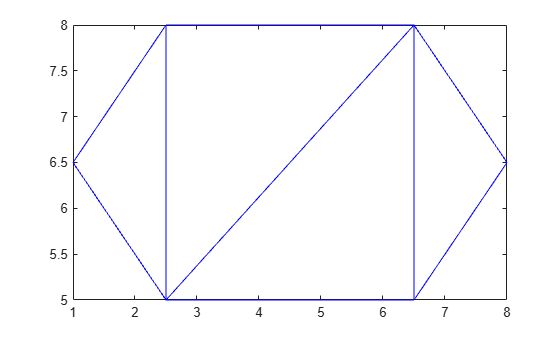cartesianToBarycentric

Convert coordinates from Cartesian to barycentric

Description

example

B = cartesianToBarycentric(TR,ID,C) returns the barycentric coordinates of the points in C relative to the triangulation object TR. Each row of C contains the Cartesian coordinates of a point with respect to the triangle or tetrahedron indexed by ID. The identification numbers of the triangles or tetrahedra in TR are the corresponding row numbers of the property TR.ConnectivityList.

Examples

collapse all

Create a triangulation from a set of points P and triangulation connectivity list T, and plot the triangulation.

P = [2.5 8.0; 6.5 8.0; 2.5 5.0; 6.5 5.0; 1.0 6.5; 8.0 6.5];
T = [5 3 1; 3 2 1; 3 4 2; 4 6 2];
TR = triangulation(T,P);
triplot(TR)Find the Cartesian coordinates of the third vertex in the first (leftmost) triangle in TR.

L = TR.ConnectivityList(1,3);
C = TR.Points(L,:)
C = 1×2

2.5000    8.0000

Convert the point C to barycentric coordinates with respect to the first triangle.

B = cartesianToBarycentric(TR,1,C)
B = 1×3

0     0     1

Input Arguments

collapse all

Triangulation representation, specified as a scalar triangulation or delaunayTriangulation object.

Data Types: triangulation | delaunayTriangulation

Triangle or tetrahedron identification, specified as a scalar or a column vector whose elements each correspond to a single triangle or tetrahedron in the triangulation object. The identification number of each triangle or tetrahedron is the corresponding row number of the ConnectivityList property.

Data Types: double

Cartesian coordinates, specified as a two-column matrix for 2-D coordinates or a three-column matrix for 3-D coordinates.

Data Types: double